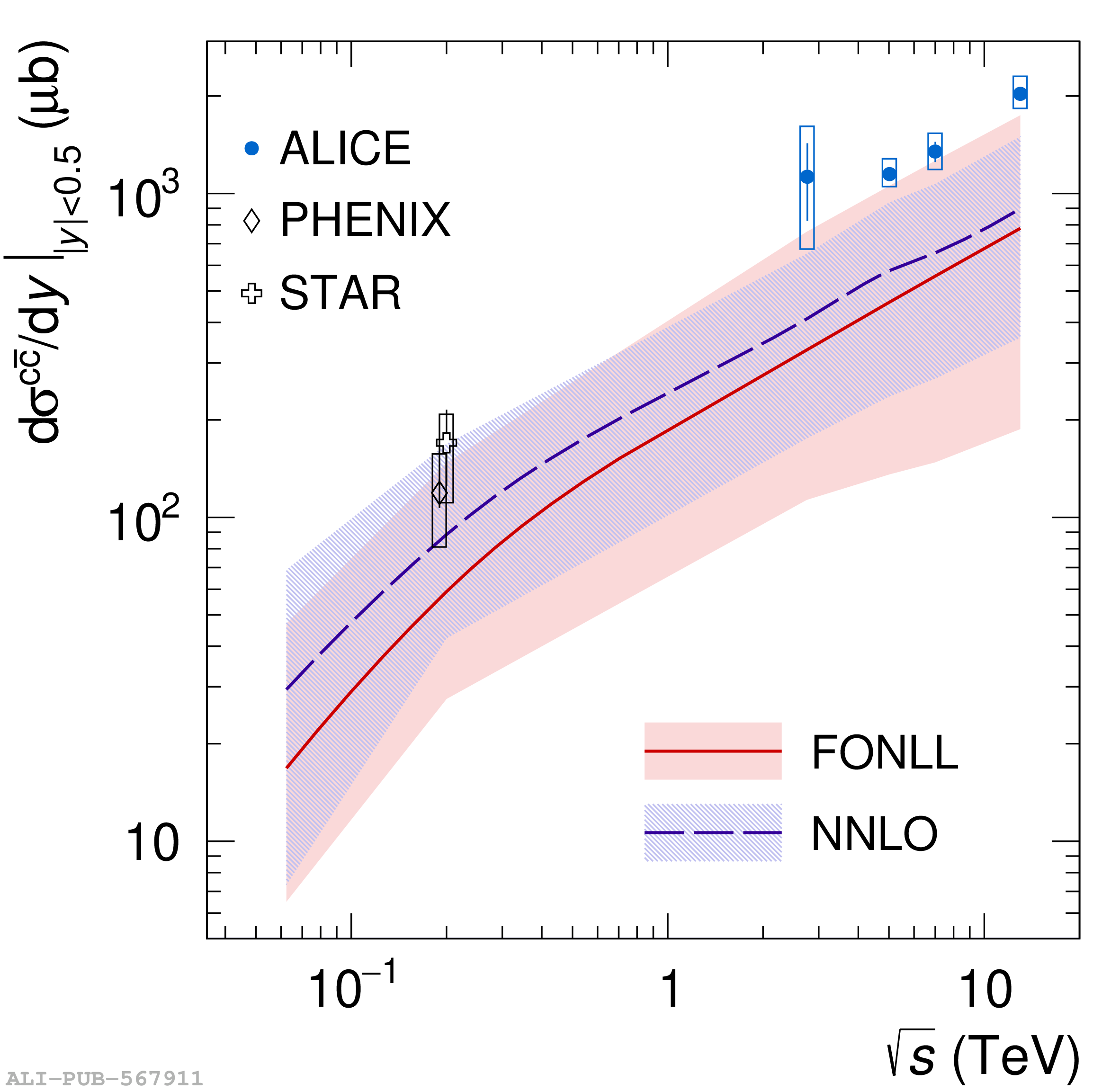# Figure 13

 Left: charm-quark fragmentation fractions at midrapidity $(|y|<0.5)$ in pp collisions at $\s=5.02$ TeV and $\s=13$ TeV compared with results in $\rm e^+e^-$ and $\rm ep$ collisions . The fragmentation fractions $f(\rm c\to h_{\rm c})$ of ${\rm J}/\psi$ mesons are multiplied by a factor 20 for better visibility. Right: $\rm c\bar{c}$ production cross section per unit of rapidity at midrapidity ($|y|<0.5$) in pp collisions as a function of $\s$. The measurements are compared with predictions from FONLL  and NNLO  calculations. The statistical and systematic uncertainties are reported as vertical bars and boxes, respectively.